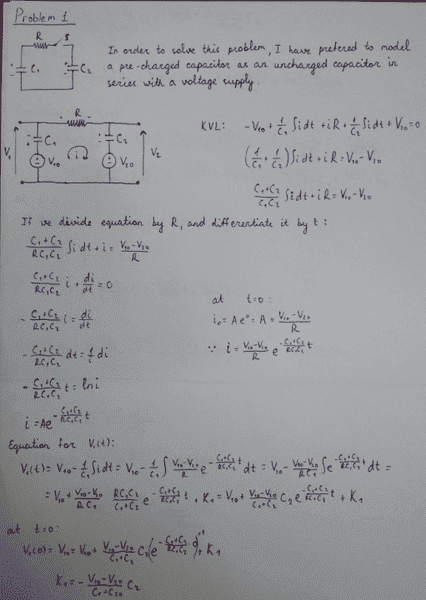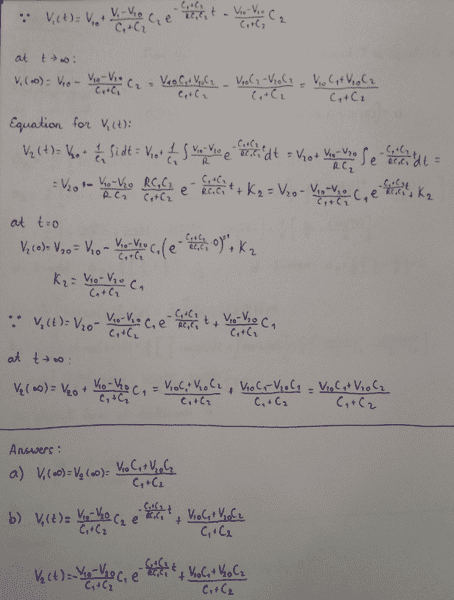# RCC circuit

New member warned about posting with no effort

## Homework Statement

The switch is closed at the moment t=0 and initial capacitor voltages are V01 and V02. How to find capacitor voltage time dependencies for both capacitors.

## The Attempt at a Solution[/B]

Last edited:

## Answers and Replies

What have you tried till now ?

It seems fine .
I also think so, but my teacher's feedback:
"Time constant and steady-state are OK but the whole solution not - when I substitute t=0 and I don't get V1(0) and V2(0).

Steady-state may be found using charge conservation law. The difference between initial condition and steady-state decays exponentially in the 1st order circuit.

So please make another (final) iteration."

I think I see a mistake - you first use indefinite integration for ' i ' as a function of ' t ' .
You don't use the limits correctly , i.e. , you don't subtract ' i ' at t = 0 .

Correct this , and then we can check if some other error creeps up .

Hope this helps .

I think I see a mistake - you first use indefinite integration for ' i ' as a function of ' t ' .
You don't use the limits correctly , i.e. , you don't subtract ' i ' at t = 0 .

Correct this , and then we can check if some other error creeps up .

Hope this helps .
I did not quiet get it. What limits should I use: t and 0?

•Qwertywerty
I did not quiet get it. What limits should I use: t and 0?
Could you explain what my teacher wanted to say by: "the whole solution not - when I substitute t=0 and I don't get V1(0) and V2(0)" and "difference between initial condition and steady-state decays exponentially in the 1st order circuit"

Could you explain what my teacher wanted to say by: "the whole solution not - when I substitute t=0 and I don't get V1(0) and V2(0)" and "difference between initial condition and steady-state decays exponentially in the 1st order circuit"
The first part - At t = 0 , by your solution , V1 is not equal to V10 , as given in the question . Same for the other capacitor's initial potential drop ( V20 ) .

The second part - This means that q ( charge on any one capacitor at time t ) would be such a function that -

q0 - q = k.ek1 , where k and k1 are some constants that will be found on solving entirely .

I think I see a mistake - you first use indefinite integration for ' i ' as a function of ' t ' .
You don't use the limits correctly , i.e. , you don't subtract ' i ' at t = 0 .

Correct this , and then we can check if some other error creeps up .

Hope this helps .
Isn't there when t=0: V1(0)=V10? Because by calculation I get the same equation.

Did you mean this? But answer doesn't change.

Mark44
Mentor
@@[email protected], please stop deleting the content of your posts. When other people have responded, deleting your posts makes it impossible to follow the discussion.